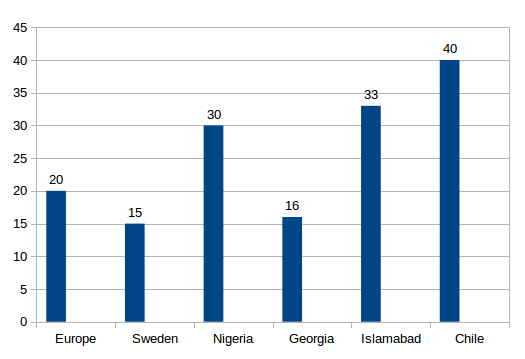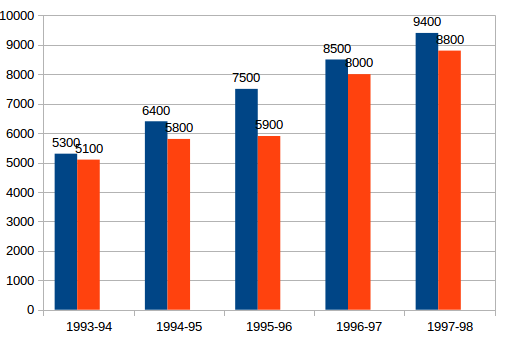## Data Interpretation For SBI PO : 631) The birth-rate of which country is 25% more than that of Georgia?

b) Chile

c) Europe

d) Nigeria

e) None of these

c) Europe

The birth rate of Georgia = 16

The birth rate of Europe = 20

So the required % increase = 20-16/16*100= 25%

Hence the birth rate of europe is 25% more than that of Georgia

2)The birth rate of India is what per cent of the birth-rate of Europe?

a) 165%

b) 155%

c) 140%

d) 100%

e) None of these

a) 165%

Birth rate of Islamabad = 33

Birth rate of Europe = 20

Required Percentage = 33 / 20*100= 165%

3) The birth-rate of Chile is how many times the birth-rate of Georgia?

a) 0.4

b) 5.2

c) 4.0

d) 2.5

e) None of these

d) 2.5

Birth rate of Chile= 40

Birth rate of Georgia = 16

Hence the birth rate of chile is 40/16 = 2.5 times that of birth rate of Georgia

4)What is the ratio of birth-rates of Islamabad to that of Sweden ?

a) 5 : 11

b) 11 : 5

c) 2 : 1

d) 1 : 2

e) None of these

b) 11 : 5

Birth rate of Islamabad = 33

Birth rate of Sweden = 15

Required Ratio = 38/15 = 11/5=11:5

5) By how much per cent is the birth-rate of Europe less than the birth-rate of Nigeria?

a) 30%

b) 33  1/3 %

c) 45%

d) 50%

e) None of these

b) 33  1/3 %

Birth rate of Europe = 20

Birth rate of Nigeria = 30

Required Percentage = 30 – 20/30*100=100/3=33 1/3%

Blue Bar: Gross traffic receipts

Orange Bar: Total expenditure.6) What is the percentage increase in the gross traffic receipts in 1995-96 as compared to 1993-94?

a) 33.9%

b) 41.5%

c) 20.7%

d) 17%

e) None of these

b) The gross traffic receipts in 1995-96 = 7500 crore and in 1993-94= 5300 crore

The required % increase = 7500-5300/5300*100=41.5%

7) If profit = gross traffic receipts— total expenditure, then in 1996-97, what percentage of gross traffic receipts is the profit made?

a) 5.9%

b) 6.4%

c) 7.2%

d) 8%

e) None of these

a)

Profit in year 1996-97 = Gross Traffic Receipt – Total Expenditure = 8500-8000 = 500 crore

Therefore Profit percent of Gross Traffic Receipt = 500/8500*100= 5.9%

8) In which year was the profit as a percentage of gross traffic receipts the highest?

a) 1997-98

b) 1996-97

c) 1995-96

d) 1994-95

e) None of these

c)

Profit as a percent of Gross Traffic Receipt in year 1997 -98 = 9400-8800/9400*100=6.38%

Profit as a percent of Gross Traffic Receipt in year 1996 -97 = 8500-8000/8500*100=5.9%

Profit as a percent of Gross Traffic Receipt in year 1995-96 = 7500-5900/7500100=21.33%

Profit as a percent of Gross Traffic Receipt in year 1994-95 = 6400-5800/6400*100=9.375%

Highest in 1995 – 96

9) In order to make a profit of 10%, what should have been the gross traffic receipts (in Rs. crores) in 1994-95, total expenditure remaining the same?

a) 5,667

b) 5,876

c) 6,444

d) 7,667

e) None of these

c)

Total Expenditure in 1994 – 95 = 5800 crore

Let the gross traffic receipt = x

So x-5800/x*100-10

-> x-5800/x=1/10

-> 10x-58000=x->9x=58000

-> x=58000/9 = 6444 crore

10) By what amount (in Rs. crores) has the expenditure increased over the period 1993-94 to 1997-98?

a) 4,100

b) 3,900

c) 3,850

d) 3,700

e) None of these